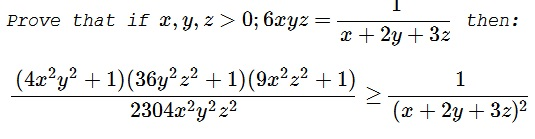# Dan Sitaru's Cyclic Inequality In Three Variables with Constraints IV

### Problem### Solution 1

First of all, we simplify the problem by replacing the variables: $a=x,\,$ $b=2y,\,$ $c=3z,\,$ which reduces the problem to

Prove that if $a,b,c\gt 0;\,abc(a+b+c)=1\,$ then:

$\displaystyle \frac{(a^2b^2+1)(b^2c^2+1)(c^2a^2+1)}{a^2b^2c^2}\geq \frac{64}{(a+b+c)^2}.$

We rewrite the inequality as

$\displaystyle \left(a^2+\frac{1}{b^2}\right)\left(b^2+\frac{1}{c^2}\right)\left(c^2+\frac{1}{a^2}\right)\geq \frac{64}{(a+b+c)^2}.$

Note that

\displaystyle \begin{align} a^2+\frac{1}{b^2} &= a^2+\frac{abc(a+b+c)}{b^2}=a^2+\frac{ac(a+b+c)}{b}\\ &=\frac{a^2b+a^2c+ac(b+c)}{b}=\frac{a^2(b+c)+ac(b+c)}{b}\\ &=\frac{a(b+c)(c+a)}{b}. \end{align}

Similarly $\displaystyle b^2+\frac{1}{c^2}=\frac{b(c+a)(a+b)}{c}\,$ and $\displaystyle c^2+\frac{1}{a^2}=\frac{c(a+b)(b+c)}{a}.$

By multiplying the three relationships,

\displaystyle \begin{align} &\Bigr(a^2+\frac{1}{b^2}\Bigr)\Bigr(b^2+\frac{1}{c^2}\Bigr)\Bigr(c^2+\frac{1}{a^2}\Bigr)\\ &\qquad\qquad\geq \frac{a(b+c)(a+c)\cdot b(a+c)(b+a)\cdot c(a+b)(c+b)}{abc}\\ &\qquad\qquad=(a+b)^2(b+c)^2(c+a)^2\\ &\qquad\qquad\overbrace{\geq}^{AM-GM}(2\sqrt{ab})^2\cdot (2\sqrt{bc})^2 \cdot (2\sqrt{ac})^2\\ &\qquad\qquad=64a^2b^2c^2=\frac{64}{(a+b+c)^2} \end{align}

The equality holds if $\displaystyle a=b=c=\frac{1}{\sqrt{3}},\,$ which follows from

$\displaystyle a\cdot a\cdot a =\frac{1}{a+a+a}\Leftrightarrow a^3=\frac{1}{3a}\Rightarrow a^4=\frac{1}{3}\Rightarrow a=\frac{1}{\sqrt{3}}.$

The equality in the original relationship holds for

$\displaystyle x=\frac{1}{\sqrt{3}}; y=\frac{1}{2\cdot\sqrt{3}}; z=\frac{1}{3\cdot\sqrt{3}}$

### Solution 2

Using the constraint, the inequality can be written as

$\displaystyle (4x^2y^2+1)(36y^2z^2+1)(9x^2z^2+1)\geq 64(6xyz)^4$

Let,

$\displaystyle x=\sqrt{\frac{ab}{c}},~2y=\sqrt{\frac{bc}{a}},~3z=\sqrt{\frac{ca}{b}}.$

Thus, the inequality and the constraint, respectively, become

$\displaystyle (a^2+1)(b^2+1)(c^2+1)\geq64(abc)^2,~ab+bc+ca=1.$

Let $p=3(abc)^{2/3}$. AM-GM gives

$\displaystyle 1=ab+bc+ca\geq3(abc)^{2/3}=p.$

The inequality can be simplified to

$\displaystyle 1+(a^2+b^2+c^2)+(a^2b^2+b^2c^2+c^2a^2)-63(abc)^2\geq 0.$

\displaystyle \begin{align} LHS &\geq 1+3(abc)^{2/3}+3(abc)^{4/3}-63(abc)^2~\text{(AM-GM)} \\ &= 1+p+\frac{p^2}{3}-\frac{7}{3}p^3\\ &= \frac{(1-p)}{3}[7(1-p)^2-20(1-p)+16] \\ &= \frac{(1-p)}{3}\left\{\left[(1-p)\sqrt{7}-\frac{10}{\sqrt{7}}\right]^2+\frac{12}{7}\right\}\geq 0, \end{align}

from the constraint.

### Acknowledgment

Dan Sitaru has kindly posted the above problem of his from the Romanian Mathematical Magazine at the CutTheKnotMath facebook page and later emailed me his solution. Solution 2 is by Amit Itagi.

A refinement of the inequality suggested by N. N. Taleb is dealt with on a separate page.